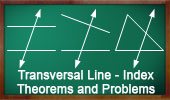Transversal Line, Theorems and Problems - Table of Content 1Ceva's Theorem. Concurrency. Interactive proof with animation. Key concept: Menelaus Theorem. Menelaus' Theorem. Interactive proof with animation and key concepts. Transversal line. Pascal's Mystic Hexagram Theorem Proof Transversal line. Pappus Theorem. Dynamic Geometry. Step-by-Step construction, Manipulation, and animation. Transversal line. Angle between two Simson Lines. Proof with animation. Transversal line. Simson Line. A proof of Simson line with animation. Transversal line. Interactive Simson Line. Dynamic Geometry. Step-by-Step construction, Manipulation, and animation. Transversal line. Routh's Theorem - Index Triangle, Cevians, Area, Ratio. Transversal line. Bottema's Theorem: Triangle and Squares with Interactive Geometry Software Step-by-Step construction, Manipulation, and animation. Monge & d'Alembert Three Circles Theorem I with Dynamic Geometry You can alter the geometric construction dynamically in order to test and prove (or disproved) conjectures and gain mathematical insight that is less readily available with static drawings by hand. Requires Java Plug-in 1.3 or higher. Please be patient while the applet loads on your computer. If you are using a dial-up connection, it may take a few minutes but is well worth the wait. Cabri, GSP, Cinderella, C.a.R. Gergonne Point Theorem. Concurrency. Interactive proof with animation. Key concept: Ceva's Theorem. Nagel Point Theorem. Proof. Interactive Gergonne Line and Nobbs Points. Dynamic Geometry. Step-by-Step construction, Manipulation, and animation. Transversal line. Newton's Theorem, Newton-Gauss Line: Complete quadrilateral theorem. Using TracenPoche Dynamic Geometry Software, Online Step-by-Step construction, manipulation, and animation. Schiffler Point: Four Euler Lines with interactive animation and manipulation. Complete Quadrilateral: Ortholine-Steiner Line. Using TracenPoche Dynamic Software Step-by-Step construction, Manipulation, and animation Go to Page: Previous | 1 | 2 | 3 | 4 | 5 | 6 | 7 | 8 | 9 | 10 | Next

 Home | Search | Geometry | Lines | Post a comment | Email | By Antonio Gutierrez Last updated: Nov 20, 2014